# Introduction

Denodo Platform allows the creation of custom functions in java for VDP and ITPilot.

Custom functions enable users to extend the set of functions available. Custom functions are implemented as Java classes included in a Jar file that is added to Virtual DataPort or to ITPilot. These custom functions can be used in the same way as every other function like MAX, MIN, SUM, etc.

Virtual DataPort also allows the creation of condition and aggregation custom functions.

Each function must be in a different Java class, but it is possible to group them together in a single Jar file. It is recommended to create custom functions using Java annotations.

We have defined a set of custom functions that could be useful in different projects. There are several blocks of functions and we classify them as:

• ITPilot custom functions:

• date

• email

• file

• string

• VDP custom functions:

• date

• Encryption

• hash

• JSON

• string

• spatial

• pivot

# ITPilot custom functions

## Date

Date-related custom functions for ITPilot.

• addday(input, increment): This function adds increment days to the input date. increment could be > or < than 0.

To use these functions, you must get a date in ITPilot, for example, input = Sat Mar 17 11:21:52 PDT 2018, and use addday in an expression as:

The result will be:

Thu Mar 18 11:21:52 PDT 2018

You can also use:

ADDDAY(input, -1) with result Tue Mar 16 11:21:52 PDT 2018

ADDDAY(input, 30) with result Fri Apr 16 11:21:52 PDT 2018

• addhour(input, increment): This function adds increment hour to the input date. increment could be > or < than 0.

To use these functions, you must get a date in ITPilot, for example, input = Sat Mar 17 11:21:52 PDT 2018, and use addhour in an expression as:

The result will be:

Sat Mar 17 12:21:52 PDT 2018

You can also use:

ADDHOUR(input, -36) with result Thu Mar 15 23:21:52 PDT 2018

ADDHOUR(input, 12) with result Sat Mar 17 23:21:52 PDT 2018

• addmillis(input, increment): This function adds increment millisecond to the input date. increment could be > or < than 0.

To use these functions, you must get a date in ITPilot, for example, input = Sat Mar 17 11:21:52 PDT 2018, and use addmillis in an expression as:

The result will be:

Sat Mar 17 11:21:52 PDT 2018

It looks the same, but if you use bigger increments
:

ADDMILLIS(input, 6000) with result Sat Mar 17 11:21:58 PDT 2018

ADDMILLIS(input, -3600000) with result Sat Mar 17 10:21:52 PDT 2018

• addminute(input, increment): This function adds increment minutes to the input date. increment could be > or < than 0.

To use these functions, you must get a date in ITPilot, for example, input = Sat Mar 17 11:21:52 PDT 2018, and use addminute in an expression as:

The result will be:

Sat Mar 17 11:21:53 PDT 2018

You can also use:

ADDMINUTE(input, -30) with result Sat Mar 17 10:51:53 PDT 2018

ADDMINUTE(input, 15) with result Sat Mar 17 11:36:53 PDT 2018

• addmonth(input, increment): This function adds increment months to the input date. increment could be > or < than 0.

To use these functions, you must get a date in ITPilot, for example, input = Sat Mar 17 11:21:52 PDT 2018, and use addmonth in an expression as:

The result will be:

Sat Apr 17 11:21:52 PDT 2018

You can also use:

ADDMONTH(input, -3) with result Thu Dec 17 11:21:52 PST 2009

ADDMONTH(input, 18) with result Sat Sep 17 11:21:52 PDT 2011

• addsecond(input, increment): This function adds increment seconds to the input date. increment could be > or < than 0.

To use these functions, you must get a date in ITPilot, for example, input = Sat Mar 17 11:21:52 PDT 2018, and use addsecond in an expression as:

The result will be:

Sat Mar 17 11:21:53 PDT 2018

You can also use:

ADDSECOND(input, -30) with result Sat Mar 17 11:21:22 PDT 2018

ADDSECOND(input, 180) with result Sat Mar 17 11:24:52 PDT 2018

• addweek(input, increment): This function adds increment weeks to the input date. increment could be > or < than 0.

To use these functions, you must get a date in ITPilot, for example, input = Sat Mar 17 11:21:52 PDT 2018, and use addweek in an expression as:

The result will be:

Sat Mar 24 11:21:52 PDT 2018

You can also use:

ADDWEEK(input, 3) with result Sat Apr 07 11:21:52 PDT 2018

ADDWEEK(input, -8) with result Sat Jan 20 11:21:52 PST 2018

• addyear(input, increment): This function adds increment years to the input date. increment could be > or < than 0.

To use these functions, you must get a date in ITPilot, for example, input = Sat Mar 17 11:21:52 PDT 2018, and use addyear in an expression as:

The result will be:

Sun Mar 17 11:21:52 PDT 2019

You can also use:

ADDYEAR(input, 3) with result Wed Mar 17 11:21:52 PST 2021

ADDYEAR(input, -8) with result Wed Mar 17 11:21:52 PDT 2010

### lastdayofmonth

• lastdayofmonth(input): This function returns a date with the last day of the month.

To use this function, you must get a date in ITPilot, for example, input = Sat Mar 17 11:21:52 PDT 2018, and use lastdayofmonth in an expression as:

LASTDAYOFMONTH(input)

The result will be:

Sat Mar 31 11:21:52 PDT 2018

### firstdayofmonth

• firstdayofmonth(input): This function returns a date with the first day of the month.

To use this function, you must get a date in ITPilot, for example, input = Sat Mar 17 11:21:52 PDT 2018, and use firstdayofmonth in an expression as:

FIRSTDAYOFMONTH(input)

The result will be:

Thu Mar 01 11:21:52 PST 2018

### getmillis

• getmillis(input): This function returns the input time value in milliseconds.

To use this function, you must get a date in ITPilot, for example, input = Thu Nov 22 17:04:37 CET 2012, and use getmillis in an expression as:

GETMILLIS(input)

The result will be:

1521282112000

### lastdayofweek

• lastdayofweek(input): This function returns a date with the last day of the week.

To use this function, you must get a date in ITPilot, for example, input = Sat Mar 17 11:21:52 PDT 2018, and use lastdayofweek in an expression as:

LASTDAYOFWEEK(input)

The result will be:

Sun Mar 11 11:21:52 PDT 2018

### firstdayofweek

• firstdayofweek(input): This function returns a date with the first day of the week.

To use this function, you must get a date in ITPilot, for example, input = Sat Mar 17 11:21:52 PDT 2018, and use firstdayofweek in an expression as:

FIRSTDAYOFWEEK(input)

The result will be:

Mon Mar 15 11:21:52 PDT 2018

### nextweekday

• nextweekday(inputDate, weekday): This function returns the next day to inputDate that is the specified week day.

To use this function, you must get a date in ITPilot, for example input = Sat Mar 17 11:21:52 PDT 2018, and use nextweekday in an expression as:

NEXTWEEKDAY(input, 1)

...where
weekday = 1 means Monday. The result will be:

Mon Mar 19 11:21:52 PDT 2018

The possible values for weekday are from
1 to 7 where 1 = Monday and 7 = Sunday
If the
inputDate is the input date week day, it returns the day of next week.

### previousweekday

• previousweekday(inputDate, weekday): This function returns the previous day to inputDate that is the specified week day.

To use this function, you must get a date in ITPilot, for example input = Sat Mar 17 11:21:52 PDT 2018, and use previousweekday in an expression as:

PREVIOUSWEEKDAY(input, 1)

...where
weekday = 1 means Monday. The result will be:

Mon Mar 12 11:21:52 PDT 2018

The possible values for weekday are from
1 to 7 where 1 = Monday and 7 = Sunday
If the
inputDate is the input date week day, it returns the day of last week.

## Email

Email-related custom functions for ITPilot:

### sendgmail

• sendgmail(user, password, from, to, subject, content): This function sends an email using a Gmail account.

This function uses user and password to authenticate in the Gmail server and sends email from the from address to the to address with subject and content. For example:

SENDGMAIL(

"one@onemachine","oh-en-ee",

"one@onemachine","two@twomachines",

"Big message","Data Virtualization is great!")

The result will be a String message:

"email sent": if the message was correctly sent.

"sending error": if the function found any problems sending the email.

Note: With some configurations, it is possible that you have to change your settings to allow less secure apps to access your account. By default it is off. This is necessary to  send mails.  Go to the "Less secure apps" section and select Turn on.

## File

This section contains file functions:

### execute

• execute(script): This function executes a script file.

• execute(String… script): This function executes a script file. It could include parameters.

To use this function, you must use a script in the function, for example: "c:\\folder\\execute.bat".

EXECUTE(script)

You can also use as many parameters as you want, for example:

EXECUTE("c:\\folder\\execute.bat", "startup")

These functions will wait for the process to finish.

NOTE: Remember use \" at the beginning and at the end if your script contains spaces.

### executeasyn

• executeasyn(script): This function executes asynchronously a script file.

• executeasyn(String… script): This function executes asynchronously a script file. It could include parameters.

To use this function, you must use a script in the function, for example: "\"C:\\Program Files\\Denodo Platform 7.0\\bin\\scheduler_startup.bat\"".

EXECUTEASYN(script)

You can also use as many parameters as you want, for example:

EXECUTEASYN("\"c:\\Program Files\\Denodo Platform 7.0\\bin\\vqlserver.bat\"", "startup")

These functions will execute the script asynchronously.

NOTE: Remember use \" at the beginning and at the end if your script contains spaces.

## String

These custom functions are the string-related custom functions:

### deletespaces

• deletespaces(input): This function deletes the spaces on a string.

This function needs a string as input, for example input = "this is the deletespaces function" the expression to use this function should be something as:

DELETESPACES(input)

and the result will be:

"thisisthedeletespacefunction"

### propercase

• propercase(input): This function returns the first letter capitalized and the rest in lower case.

This function needs a string as input, for example if input = "CALIFORNIA" and using the following expression:

PROPERCASE(input)

...and the result will be:

"California"

This will also be the result for
"CALIFORNIA", "california", "cALIFORNIA" or any other combination of upper and lower case.

### concatlist

• concatlist(values): This function appends the values in the list using space as separator.

• concatlist(separator, values): This function appends the values in the list using a separator character.

To use these functions, you must get a list of string in ITPilot, for example, values = "this", "is", "the", "concatlist", "function",  and use concatlist in an expression as:

CONCATLIST(values)

The result will be:

"this is the concatlist function"

You can also use:

CONCATLIST(",", values)

with result

"this,is,the,deletespace,function"

### startwith

• startwith(input, start): This function returns true if the input string starts with start string.

To use this function, you must get two strings in ITPilot, for example, input = "this is the concatlist function" and value = "this", and use startwith in an expression as:

STARTWITH("this is the concatlist function", "this")

The result will be:

true

### endwith

• endwith(input, end): This function returns true if the input string ends with end string.

To use this function, you must get two strings in ITPilot, for example, input = "this is the concatlist function" and value = "on", and use endwith in an expression as:

ENDWITH("this is the concatlist function", "on")

The result will be:

true

# VDP custom functions

## Date

Date-related custom functions for VDP not included in the installation of Denodo Platform.

• addmillis(input, increment): This function adds increment milliseconds to the input date. increment could be > or < than 0.

To use these functions, you must get a date in VDP, for example, input = Sat Mar 17 11:21:52 PDT 2018, and use addmillis in an expression as:

The result will be:

Sat Mar 17 11:21:52 PDT 2018

It looks the same, but if you use bigger increments:

ADDMILLIS(input, 6000) with result Sat Mar 17 11:21:58 PDT 2018

ADDMILLIS(input, -3600000) with result Sat Mar 17 10:21:52 PDT 2018

The function addmillis will be delegated to the data source when the date comes from:

• MySQL 4 and 5.
• Oracle 9i, 10g and 11g.
• MS SQLServer 8, 2005, 2008, 2012.
• Db2 11
• Denodo Virtual DataPort 7.0 and 8.0.

### combine_date_time

• combine_date_time(date, time): This function combines the date (year, month and day of month) and the time (hour, minute, second and millisecond) of two datetime inputs into one that represents the data coming from both.

This is especially useful when the source has differentiated data types for dates and times, allowing to combine them.

This function will not be delegated to the source.

### get_time_from_millis

• gettimefrommillis(long): This function converts milliseconds to date

To use these functions you have to introduce a date expressed in milliseconds from Epoch time (1 January 1970 UTC).

GETTIMEFROMMILLIS(0)  with result Thu Jan 01 01:00:00 CET 1970

GETTIMEFROMMILLIS(1413882867000) with result  Tue Oct 21 11:14:27 CEST 2014

The function gettimefrommillis will be delegated to the data source when the date comes from:

• Oracle 9i, 10g and 11g.
• Denodo Virtual DataPort 7.0 and 8.0.

### getweeksbetween

• getweeksbetween(startdate, enddate): This function returns the number of weeks between two dates.

To use this function, you must get two dates in VDP, for example, startdate = Thu Aug 04 12:59:38 CEST 2016, and enddate= Tue Aug 16 02:46:18 CEST 2016.

The result will be:

1

This function is delegated to the following databases, in addition you can see its equivalences:

 Versions Equivalences Mysql All FLOOR(DATEDIFF(DATE(\$1),DATE(\$0) )/7) ORACLE 9i, 10g, 11g, 12c TRUNC((CAST(\$0 as DATE)-CAST( \$1 as DATE))/7) SQL SERVER All DATEDIFF(week, \$0, \$1) DB2 11 WEEKS_BETWEEN(\$0, \$1)

It is also delegated to Denodo Virtual DataPort 7.0 and 8.0.

## Encryption

These custom functions are the encryption-related custom functions.

### encrypt

This function uses random salts and initialization vectors (IV). This is why we strongly recommend the use of encrypt function, (instead of encypt_fixed), as it does ensure that encryption is not deterministic by using some initial randomness.

• encrypt(password, input): This function takes a text as input parameter and encrypts the text using the password provided as the first argument. The encryption algorithm used is PBEWithMD5AndDES.

• encrypt(algorithm, password, input): This function takes a text as input parameter and encrypts the text using the password provided as second argument and the encryption algorithm provided as first argument.

The encryption algorithm has to refer to a PBE encryption algorithm and be supported by the default JCE of the Denodo Platform JRE. The list is:

PBEWITHHMACSHA1ANDAES_128, PBEWITHHMACSHA1ANDAES_256, PBEWITHHMACSHA224ANDAES_128, PBEWITHHMACSHA224ANDAES_256, PBEWITHHMACSHA256ANDAES_128, PBEWITHHMACSHA256ANDAES_256, PBEWITHHMACSHA384ANDAES_128, PBEWITHHMACSHA384ANDAES_256, PBEWITHHMACSHA512ANDAES_128, PBEWITHHMACSHA512ANDAES_256, PBEWITHMD5ANDDES, PBEWITHMD5ANDTRIPLEDES, PBEWITHSHA1ANDDESEDE, PBEWITHSHA1ANDRC2_128, PBEWITHSHA1ANDRC2_40, PBEWITHSHA1ANDRC4_128, PBEWITHSHA1ANDRC4_40

Algorithm names follow the convention: PBEWith<digest>And<encryption>. And the recommended one is: PBEWithHMACSHA512AndAES_256.

• encrypt(provider, algorithm, password, input): This function takes a text as input parameter and encrypts the text using the password provided as second argument, the encryption algorithm provided as second argument, the function will use the implementation provided by the provider specified as first argument. The encryption algorithm has to be supported by the provider and the additional provider has to be registered as part of the Denodo Platform JRE.

To use these functions, you must get a text in VDP, for example, input = "to be or not to be", password = "mypassword" and use encrypt in an expression as:

The result will be:

"GTkGr+NeYXss3rBP2BD81P7AC/buCkF2+KTJBi/sdhQ="

You can also use:

with result

"Pbm++23/+Mh+nXzp+ayfbo9/WwqEXZoiYW5VoeBHcN0="

Or:

with result

"9KPcRLxcPuKlowYdZsIDi6s8YhLwYD4//+fsTEFVdT2tDJ+AuwOPDDnnjiZRvWEK"

These functions will not be delegated to the source.

### decrypt

This function decodes messages encrypted using the encrypt function.

• decrypt(password, input): This function takes a text as input parameter and decrypts the text using the password provided. The encryption algorithm used is PBEWithMD5AndDES.

• decrypt(algorithm, password, input): This function takes a text as input parameter and decrypts the text using the password and the encryption algorithm provided. The encryption algorithm has to be supported by the default JCE of the Denodo Platform JRE.

• decrypt(provider,algorithm, password, input): This function takes a text as input parameter and decrypts the text using the password and the encryption algorithm provided. The function will use the implementation provided by the provider specified as the first argument. The encryption algorithm has to be supported by the provider and the additional provider has to be registered as part of the Denodo Platform JRE.

To use these functions, you must get a text in VDP, for example, input = "GTkGr+NeYXss3rBP2BD81P7AC/buCkF2+KTJBi/sdhQ=", password = "mypassword" and use decrypt in an expression as:

The result will be:

"to be or not to be"

You can also use:

'Pbm++23/+Mh+nXzp+ayfbo9/WwqEXZoiYW5VoeBHcN0=')

with result

"to be or not to be"

Or:

with result

"to be or not to be"

These functions will not be delegated to the source.

### encrypt_fixed

If you need identical inputs to give identical ciphertexts you can use the encrypt_fixed function. This function uses fixed salts and initialization vectors (IV) provided by the user, unlike the encrypt function that uses random salts and IVs.

We strongly recommend the use of encrypt function, (instead of encypt_fixed), as it does ensure that encryption is not deterministic by using some initial randomness.

• encrypt_fixed(iv, salt, password, input): This function takes a text as input parameter and encrypts the text using the password provided. The encryption algorithm used is PBEWithMD5AndDES.

Note that you have to use the same IV and salt for encryption as well as decryption. For security reasons for each encryption you should use a new IV and a new salt. They should be a multiple of the algorithm block size. Typical block sizes are 8 bytes or 16 bytes.

• encrypt_fixed(iv, salt, algorithm, password, input): This function takes a text as input parameter and encrypts the text using the password and the encryption algorithm provided.

The encryption algorithm has to refer to a PBE encryption algorithm and be supported by the default JCE of the Denodo Platform JRE. The list is:

PBEWITHHMACSHA1ANDAES_128, PBEWITHHMACSHA1ANDAES_256, PBEWITHHMACSHA224ANDAES_128, PBEWITHHMACSHA224ANDAES_256, PBEWITHHMACSHA256ANDAES_128, PBEWITHHMACSHA256ANDAES_256, PBEWITHHMACSHA384ANDAES_128, PBEWITHHMACSHA384ANDAES_256, PBEWITHHMACSHA512ANDAES_128, PBEWITHHMACSHA512ANDAES_256, PBEWITHMD5ANDDES, PBEWITHMD5ANDTRIPLEDES, PBEWITHSHA1ANDDESEDE, PBEWITHSHA1ANDRC2_128, PBEWITHSHA1ANDRC2_40, PBEWITHSHA1ANDRC4_128, PBEWITHSHA1ANDRC4_40

Algorithm names follow the convention: PBEWith<digest>And<encryption>. And the recommended one is: PBEWithHMACSHA512AndAES_256.

Note that you have to use the same IV and salt for encryption as well as decryption. For security reasons for each encryption you should use a new IV and a new salt. They should be a multiple of the algorithm block size. Typical block sizes are 8 bytes or 16 bytes.

• encrypt_fixed(iv, salt, provider, algorithm, password, input): This function takes a text as input parameter and encrypts the text using the password and the encryption algorithm provided. The function will use the implementation provided by the provider specified. The encryption algorithm has to be supported by the provider and the additional provider has to be registered as part of the Denodo Platform JRE.

Note that you have to use the same IV and salt for encryption as well as decryption. For security reasons for each encryption you should use a new IV and a new salt. They should be a multiple of the algorithm block size. Typical block sizes are 8 bytes or 16 bytes.

To use these functions, you must get a text in VDP, for example, input = "my tailor is rich", password = "mypassword"  and use encrypt in an expression as:

The result will be:

"S5EkDVJgWkiqSLmSZDBl7C12rAib1ID3"

You can also use:

with result

"fOKIdP8Z+4sFx83pIQFFBEOgr+KnZENC"

Or:

with result

"TGSYjDjPgoCL44eogwg7xJ1DkRMAwFRiw1H336MtwGk="

These functions will not be delegated to the source.

### decrypt_fixed

This function decodes messages encrypted using the encrypt_fixed function.

• decrypt_fixed(iv, salt, password, input): This function takes a text as input parameter and decrypts the text using the password provided. The encryption algorithm used is PBEWithMD5AndDES.

Note that you have to use the same IV and salt for encryption as well as decryption.

• decrypt_fixed(iv, salt, algorithm, password, input): This function takes a text as input parameter and decrypts the text using the password and the encryption algorithm provided. The encryption algorithm has to be supported by the default JCE of the Denodo Platform JRE.

Note that you have to use the same IV and salt for encryption as well as decryption.

• decrypt(provider,algorithm, password, input): This function takes a text as input parameter and decrypts the text using the password and the encryption algorithm provided. The function will use the implementation provided by the provider specified as the first argument. The encryption algorithm has to be supported by the provider and the additional provider has to be registered as part of the Denodo Platform JRE.

Note that you have to use the same IV and salt for encryption as well as decryption.

To use these functions, you must get a text in VDP, for example, input = "uz8aMn8DAPORNzt5em+NP50qWT+ndsuj1DdWHZ1gweg=", password = "pass" and use decrypt in an expression as:

The result will be:

"my tailor is rich"

You can also use:

with result

"my tailor is rich"

Or:

with result

"my tailor is rich"

These functions will not be delegated to the source.

## HASH

### hash_function

• hash_function(text, hash_algorithm): Returns a hex-encoded string containing the N-bit hash_algorithm  message digest. hash_algorithm valid values are: md5, sha1, sha256 and sha512.

Example:

select hash_function('hello','SHA256') from dual();

The result will be:

2CF24DBA5FB0A30E26E83B2AC5B9E29E1B161E5C1FA7425E73043362938B9824

This function is delegated to the following databases: Oracle, MySQL, Snowflake, Db2 11 and  Denodo Virtual DataPort 7.0 and 8.0.

## JSON

These custom functions are the JSON-related custom functions.

These functions only are distributed within the xtrafuncs jar for Denodo 7.0 version. In the 8.0 version they are included “out of the box” since the denodo-v80-update-20220728.

### complex_type_to_json

• complex_type_to_json(complex_type, array_name): This function converts an VDP  array to a JSON text.

This function needs an VDP array and a string as input parameters.

For example, if you execute the following query:

select complex_type_to_json({ROW( 'George', 'Washington', '1732-02-22', ROW( '3200 Mount Vernon Memorial Highway', 'Mount Vernon', 'Virginia', 'United States' ))}, 'values') from dual();

the result will be the following JSON text:

{"values":[{"value":"George","value1":"Washington","value2":"1732-02-22","value3":{"value":"3200 Mount Vernon Memorial Highway","value1":"Mount Vernon","value2":"Virginia","value3":"United States"}}]}

• complex_type_to_json(complex_type): This function converts a VDP register to a JSON text.

This function needs a VDP register as input parameter.

Example:

Imagine you have a VDP view called usa_president with the following schema: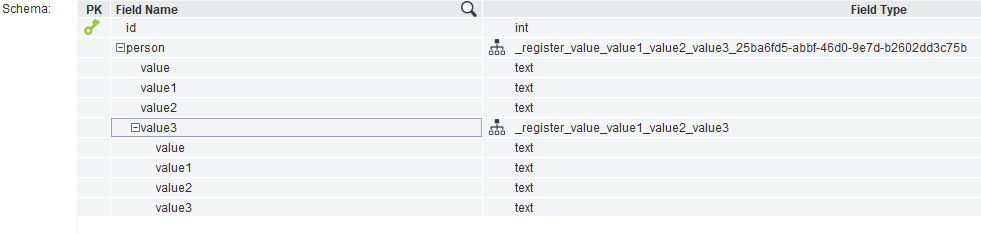If you execute the following query:

select complex_type_to_json(person) from usa_president

the result will be the following JSON text:

{"value":"George","value1":"Washington","value2":"1732-02-22","value3":{"value":"3200 Mount Vernon Memorial Highway","value1":"Mount Vernon","value2":"Virginia","value3":"United States"}}

### json_to_complex_type

• json_to_complex_type(json_text, json_schema): This function converts a JSON expression to a register complex type.

This function needs two strings as parameters: the JSON text and its JSON schema representation.

Important: The JSON schema must not use neither patternProperties nor itemPrefix keys. Use properties and items keys instead. The \$schema valid versions are :  2019-19, V7, V6 e V4.

For example, if you execute the following query:

select json_to_complex_type('{

"firstName": "John",

"lastName": "Doe",

"age": 21

}

',

'{

"\$id": "https://example.com/person.schema.json",

"\$schema": "https://json-schema.org/draft/2019-09/schema",

"title": "Person",

"type": "object",

"properties": {

"firstName": {

"type": "string"

},

"lastName": {

"type": "string"

},

"age": {

"type": "integer"

}

}

}') as result from dual();

the result will be: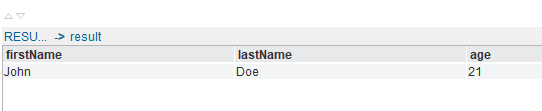### jsonpath

• jsonpath(json_value, json_path_expression): This function returns the nodes from an JSON document selected by an JSON path expression

This function needs two strings as input parameters: the JSON text and the JSON path expression.

For example, if you execute the following query:

select jsonpath('{

"store": {

"book": [

{

"category": "reference",

"author": "Nigel Rees",

"title": "Sayings of the Century",

"price": 8.95

},

{

"category": "fiction",

"author": "Evelyn Waugh",

"title": "Sword of Honour",

"price": 12.99

},

{

"category": "fiction",

"author": "Herman Melville",

"title": "Moby Dick",

"isbn": "0-553-21311-3",

"price": 8.99

},

{

"category": "fiction",

"author": "J. R. R. Tolkien",

"title": "The Lord of the Rings",

"isbn": "0-395-19395-8",

"price": 22.99

}

],

"bicycle": {

"color": "red",

"price": 19.95

}

},

"expensive": 10

}', '\$.store.book') as json_path_results from dual();

the result will be:

{"category":"reference","author":"Nigel Rees","title":"Sayings of the Century","price":8.95}

These functions will not be delegated to the source.

## String

These custom functions are the string-related custom functions:

### deletespaces

• deletespaces(input): This function deletes the spaces on a string.

This function needs a string as input, for example input = "this is the deletespace function" the expression to use this function should be something as:

DELETESPACES(input)

and the result will be:

"thisisthedeletespacefunction"

This function is delegated to the following databases, in addition you can see its equivalences:

 Versions Equivalences Mysql All REPLACE(\$0,'' '','''') ORACLE All REPLACE(\$0,'' '','''') SQL SERVER All REPLACE(\$0,'' '','''') Db2 10, 11 REPLACE(\$0,'' '','''') Denodo Virtual DataPort 7.0, 8.0 deletespaces(\$0)

### propercase

• propercase(input): This function returns the first letter capitalized and the rest in lower case.

This function needs a string as input, for example input = "CALIFORNIA" and using the following expression:

PROPERCASE(input)

and the result will be:

"California"

This will be the result for "CALIFORNIA", "california", "cALIFORNIA" or any other combination of upper and lower case.

This function is delegated to the following databases, in addition you can see its equivalences:

 Versions Equivalences Mysql All CONCAT(UCASE(SUBSTRING(\$0, 1,1)), LOWER(SUBSTRING(\$0, 2))) ORACLE All CONCAT(UPPER(SUBSTR(\$0,1,1)),LOWER(SUBSTR(\$0,2))) SQL SERVER All UPPER(SUBSTRING(\$0,1,1))+ LOWER(SUBSTRING (\$0,2,DATALENGTH(\$0))) Db2 10, 11 CONCAT(UPPER(SUBSTR(\$0, 1, 1)),LOWER(SUBSTR(\$0, 2))) Denodo Virtual DataPort 7.0, 8.0 propercase(\$0)

### concatlist

• concatlist(values): This function concats the values in the array using space as separator.

• concatlist(separator, values): This function concats the values in the array using a separator character.

To use these functions, you must get an Array (JDBC Array) in VDP, for example, values = Array["this", "is", "the", "concatlist", "function"], and use concatlist in an expression as:

CONCATLIST(values)

The result will be:

"this is the concatlist function"

You can also use:

CONCATLIST(",", values)

with result

"this,is,the,deletespace,function"

This function is delegated to Denodo Virtual DataPort 7.0 and 8.0.

### startwith

• startwith(input, start): This function returns true if the input string starts with start string.

To use this function, you must get two strings in VDP, for example, input = "this is the concatlist function" and value = "this", and use start with in an expression as:

STARTWITH("this is the concatlist function", "this")

The result will be:

true

This function is delegated to the following databases, in addition you can see its equivalences:

 Versions Equivalences Mysql All CASE WHEN (\$0 LIKE CONCAT(\$1,''%'')) THEN 'true' ELSE 'false' END ORACLE 9i,10g,11g,12 CASE WHEN (\$0 LIKE CONCAT(\$1,''%'')) THEN 1 ELSE 0 END SQL SERVER All CASE WHEN \$0 LIKE (\$1+''%'') THEN 1 ELSE 0 END Db2 10, 11 CASE WHEN (\$0 LIKE CONCAT(\$1,''%'')) THEN 1 ELSE 0 END Denodo Virtual DataPort 7.0, 8.0 STARTWITH(\$0,\$1)

### endwith

• endwith(input, end): This function returns true if the input string ends with end string.

To use this function, you must get two strings in VDP, for example, input = "this is the concatlist function" and value = "on", and use end with in an expression as:

ENDWITH("this is the concatlist function", "on")

The result will be:

true

This function is delegated to the following databases, in addition you can see its equivalences:

 Versions Equivalences Mysql All CASE WHEN (\$0 LIKE CONCAT(''%'',\$1)) THEN 'true' ELSE 'false' END ORACLE 9i,10g,11g,12 CASE WHEN (\$0 LIKE CONCAT(''%'',\$1)) THEN 1 ELSE 0 END SQL SERVER All CASE WHEN \$0 LIKE (''%''+\$1) THEN 1 ELSE 0 END Db2 10, 11 CASE WHEN \$0 LIKE CONCAT(''%'',\$1) THEN 1 ELSE 0 END Denodo Virtual DataPort 7.0, 8.0 ENDWITH(\$0, \$1)

For example:

The result will be:

"A"

This function is delegated to the following databases, in addition you can see its equivalences, with two or three parameters respectively:

For example:

The result will be:

"0087"

This function is delegated to the following databases, in addition you can see its equivalences, with two or three parameters respectively:

### printf

• printf(expression, value): Performs a String.format(...) formatting operation.

• printf(locale, expression, value): Performs a String.format(...) formatting operation.

For example:

PRINTF('Item unavailable %1\$s', item_name)

This function is delegated to Denodo Virtual DataPort 7.0 and 8.0.

### base64_to_base10

• base64_to_base10(text): Convert a String from base64 to base10.

For example:

BASE64_TO_BASE10('russellwhyte')

The result will be:

35423280641357683489288844389

This function is delegated to Denodo Virtual DataPort 7.0 and 8.0.

• trimleading(pattern, text): remove pattern from the front  of string. If the pattern is repeated in the front, all the repetitions will be removed.

For example:

The result will be:

denodo

This function is delegated to the following databases, in addition you can see its equivalences:

 Versions Equivalences Mysql All TRIM(LEADING \$0 FROM \$1) ORACLE All TRIM(LEADING \$0 FROM \$1) Db2 10, 11 TRIM(LEADING \$0 FROM \$1) Denodo Virtual DataPort 7.0, 8.0 TRIMLEADING(\$0,\$1)

* Oracle only supports a pattern of one character.

### trimtrailing

• trimtrailing(pattern, text): remove pattern from the end of string. If the pattern is repeated in the end, all the repetitions will be removed.

For example:

TRIMTRAILING('*','denodo***')

The result will be:

denodo

This function is delegated to the following databases, in addition you can see its equivalences:

 Versions Equivalences Mysql All TRIM(TRAILING \$0 FROM \$1) ORACLE All TRIM(TRAILING \$0 FROM \$1) Db2 10, 11 TRIM(TRAILING \$0 FROM \$1) Denodo Virtual DataPort 7.0, 8.0 TRIMTRAILING(\$0,\$1)

*Oracle only supports a pattern of one character.

### trimboth

• trimboth(pattern, text): remove pattern from the front and the of string. If the pattern is repeated in the front and the end, all the repetitions will be removed.

For example:

TRIMBOTH('*','****denodo****')

The result will be:

denodo

This function is delegated to the following databases, in addition you can see its equivalences:

 Versions Equivalences Mysql All TRIM(BOTH \$0 FROM \$1) ORACLE All TRIM(BOTH \$0 FROM \$1) Db2 10, 11 TRIM(BOTH \$0 FROM \$1) Denodo Virtual DataPort 7.0, 8.0 TRIMBOTH(\$0,\$1)

*Oracle only supports a pattern of one character.

### base64_to_hex

• base64_to_hex(text): Convert a String from base64 to hexadecimal (hex) or base16.

For example:

BASE64_TO_HEX('VGhlIHJhaW4gaW4gc3BhaW4gaXMgYWx3YXlzIGluIHRoZSBwbGFpbg==')

The result will be:

546865207261696e20696e20737061696e20697320616c7761797320696e2074686520706c61696e

This function is delegated to Denodo Virtual DataPort 7.0 and 8.0.

### hex_to_base64

• hex_to_base64(text): Convert a String from hexadecimal (hex) or base16 to base64.

For example:

HEX_TO_BASE64('546865207261696e20696e20737061696e20697320616c7761797320696e2074686520706c61696e')

The result will be:

VGhlIHJhaW4gaW4gc3BhaW4gaXMgYWx3YXlzIGluIHRoZSBwbGFpbg==

This function is delegated to Denodo Virtual DataPort 7.0 and 8.0.

## Spatial

These custom functions are the spatial-related custom functions. These functions use the JTS Topology Suite library and support the following spatial data types: point, multipoint, linestring, multilinestring, polygon and multipolygon.

The geometric operations of this section work by default on a two-dimensional cartesian plane. There are several types of functions: constructor, editor, relationship or measurement. In addition there is a special function to transform a geometry into a different spatial reference. And among the measurement functions there are some functions with  the _meters suffix that make transformations underneath (if needed) to give the result in meters.

ST_transform, ST_buffer_meters and the measurement functions with the _meters suffix take into consideration the coordinate reference system (CRS) of the geometry in order to compute a result. For this, these functions will ask for a code that identifies the CRS of the geometry of the input, or two codes if there are two geometries being input to the function. Note that the specific CRS being used might --among other things-- determine the order of the axis in the geometry. The CRS codes need to include the authority (usually EPSG). Examples of codes:

EPSG:1234

AUTO:42001

You must take into account that in the geographic CRS, the (latitude, longitude) axis order has been widely used by geographers and pilots for centuries. However software developers tend to consistently use the (x, y) order for every kind of CRS. Those different practices resulted in contradictory definitions of axis order. That is why, to avoid ambiguity when specifying spatial reference systems in some scenarios, you may need to force (longitude, latitude) axis order. To address this issue, you can add the :XY suffix in the code as you can see in the example below:

EPSG:4326:XY

Note that if you do not add the :XY suffix it means that the axis order will be determined by the system default option (not “latitud first”).

All the names of the spatial functions have the ST_ (Spatial Type) prefix. Most of these functions have two versions: one in which geometries are represented as well-known binary (wkb) and another one in which they are represented in well-known text (wkt) format.

An error in these functions will return null as a result, in order to keep consistency with other VQL functions. The cause and the trace of the error will be output through VDP’s log.

### st_geom_to_struct

• st_geom_to_struct(wkb): This function parses a blob that represents a geometry and converts it into a structural representation  of the geometry in terms of register and arrays.

• st_geom_to_struct(wkt): This function parses a text that represents a geometry and converts it into a structural representation of the geometry in terms of register and arrays.

The structural representation of the geometry is a VDP register (struct) with the following fields:

• type: the geometry type (point, linestring, polygon…).

• bounding_box: a register with the coordinates of the minimum rectangle that contains the geometry.

• point: a register with the coordinates x and y of the point, if the type field states that the geometry is a point.

• linestring: an array with the points that form the linestring, if the type field states that the geometry is a linestring.

• polygon: a register defined as the exterior linestring (a.k.a. shell) and an array with the holes (linestrings) in the polygon, if the type field states that the geometry is a polygon.

• multipoint: an array with all its points, if the type field states that the geometry is a multipoint.

• multilinestring: an array with all its linestrings, if the type field states that the geometry is a multilinestring.

• multipolygon: an array with all its polygons, if the type field states that the geometry is a multipolygon.

This function can receive a string as input. The string must express a geometry in the Well-Known Text (wkt) format. For example, with input = 'LINESTRING(1 1, 5 5, 10 10, 20 20)', the expression to use this function should be something as:

st_geom_to_struct(input)

...and the result would be:

{ type = 'LineString',

bounding_box = {

max_x = 20.0,

max_y = 20.0,

min_x = 1.0,

min_y = 1.0 },

point = null,

linestring = Array [

{ x = 1.0, y = 1.0 },

{ x = 5.0, y = 5.0 },

{ x = 10.0, y = 10.0 },

{ x = 20.0, y = 20.0 } ],

polygon = null,

multipoint = null,

multilinestring = null,

multipolygon = null }

This function can also receive a blob as input. The blob must express a geometry in the Well-Known Binary (wkb) format.

### st_distance

• st_distance(wkb1, wkb2)/st_distance(wkt1, wkt2): This function returns the minimum distance between the geometry wkb1 and the geometry wkb2.

For example:

ST_DISTANCE(

'LINESTRING (30 10, 10 30, 40 40)',

'LINESTRING (70 10, 50 30, 80 40)')

The result will be:

14.14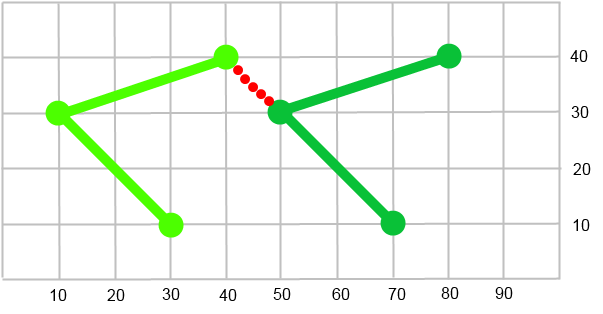In this example, the minimum distance is represented by the length of the red dotted line.

### st_distance_meters

• st_distance_meters(wkb1, code1, wkb2, code2)/st_distance_meters(wkt1, code1, wkt2, code2): This function returns the minimum distance between the geometry wkb1 and the geometry wkb2 expressed in meters. The codes identify the CRS of each geometry.

If any of the CRS of the inputs are different to WGS84, then they are projected to WGS84, and when the two geometries are in WGS84, the orthodromic distance between the two geometries is calculated.

For example:

ST_DISTANCE_METERS(

'POINT(43.37 -8.41)','EPSG:4326',

'POINT(43.50 -8.22)','EPSG:4326')

ST_DISTANCE_METERS (

'POINT(547800.41 4802073)','EPSG:32629',

'POINT (563058.68 4816636.85)','EPSG:32629')

These examples are equivalents, their operation is on the two same points, expressed in WGS84 and in UTM zone 29N respectively, and the result will be:

21100.76

Note: The two following queries are not equivalent, because the ST_distance_meters query calculates the orthodromic distance and the query of ST_distance simply calculates the distance in the Cartesian plane. Thus, in the result there is a small variation. In this case, from 21100.76 meters of the first query to the 21604.60 meters of the other query:

ST_DISTANCE_METERS(

'POINT(43.37 -8.41)','EPSG:4326 ',

'POINT(43.50 -8.22)','EPSG:4326')

ST_DISTANCE(

ST_TRANSFORM(

'POINT(43.37 -8.41)','EPSG:4326 ','AUTO:42001,8,43'),

ST_TRANSFORM(

'POINT(43.50 8.22)','EPSG:4326','AUTO:42001,8,43'))

The function st_distance_meters will be delegated to the data source when the date comes from:

• Snowflake.

### st_equals

• st_equals(wkb1, wkb2)/st_equals(wkt1, wkt2): This function returns true if the given geometries represent the same one. Directionality is ignored. This function supports all the spatial data types.

For example:

ST_EQUALS(

'LINESTRING (30 10, 10 30, 40 40)',

'LINESTRING (70 10, 50 30, 80 40)')

The result will be:

false

### st_disjoint

• st_disjoint(wkb1, wkb2)/st_disjoint(wkt1, wkt2): This function returns true if the given geometries do not have a point in common. This function supports all the spatial data types.

For example:

ST_DISJOINT('POINT (0 0)', 'LINESTRING (3 1, 1 3)')

The result will be:

true

The function st_disjoint will be delegated to the data source when the date comes from:

• Snowflake.

### st_intersects

• st_intersects(wkb1, wkb2)/st_intersects(wkt1, wkt2): This function returns true if the intersection of the given geometries does not result in an empty set. This function supports all the spatial data types.

For example:

ST_INTERSECTS('POINT (0 0)', 'LINESTRING (3 1, 1 3)')

The result will be:

false

The function st_intersects will be delegated to the data source when the date comes from:

• Snowflake.

### st_touches

• st_touches(wkb1, wkb2)/st_touches(wkt1, wkt2): This function returns true if  the given geometries have at least one point in common, but their interiors do not have any points in common. This function applies to all possible relationships between the different spatial data types except the pair point with point.

For example:

ST_TOUCHES('LINESTRING (0 0, 2 2, 0 3)','POINT (2 2)')

The result will be:

false

### st_crosses

• st_crosses(wkb1, wkb2)/st_crosses(wkt1, wkt2): This function returns true if the given geometries have some interior points in common, but not all of them. This function supports all the spatial data types.

For example:

ST_CROSSES(

'LINESTRING(3 1, 1 1, 1 3)',

'LINESTRING(-3 1, 2 2, -1 3)')

The result will be:

true

### st_within

• st_within(wkb1, wkb2)/st_within(wkt1, wkt2): This function returns true if the geometry wkb1 is completely inside geometry wkb2. This function supports all the spatial data types.

For example:

ST_WITHIN('LINESTRING(0 0, 0 1)',ST_BUFFER('POINT(0 0)',3))

The result will be:

true

The function st_within will be delegated to the data source when the date comes from:

• Snowflake.

### st_contains

• st_contains(wkb1, wkb2)/st_contains(wkt1, wkt2): This function returns true if the geometry wkb2 is completely contained by the geometry wkb1. This function supports all the spatial data types.

For example:

ST_CONTAINS('POLYGON ((0 0, 0 2, 2 2, 2 0, 0 0))','POINT (1 1)')

The result will be:

true

The function st_contains will be delegated to the data source when the date comes from:

• Snowflake.

### st_overlaps

• st_overlaps(wkb1, wkb2)/st_overlaps(wkt1, wkt2): This function returns true if  the given geometries share space, are of the same dimension, but are not completely contained by each other. This function supports all the spatial data types.

For example:

ST_OVERLAPS(

'LINESTRING (0 0, 2 2, 2 4, 0 6)',

'LINESTRING (0 6, 2 2, 2 4, 6 6)')

The result will be:

true

### st_relate

• st_relate(wkb1, wkb2, matrixPattern)/st_relate(wkt1, wkt2, matrixPattern): This function returns true if the first geometry is spatially related to the second geometry, by testing for intersections between the interior, boundary and exterior of wkb1 and wkb2 as specified by the values in the matrixPattern. The matrixPattern is a 9-character string that represents a matrix in the dimensionally-extended 9 intersection model (DE-9IM). Each character represents the type of intersection allowed at one of the nine intersections between the two geometries(interior, boundary and exterior). This function supports all the spatial data types.

For example:

ST_RELATE('POINT(3 3)', ST_BUFFER('POINT(0 0)',3),'FF0FFF212')

The result will be:

true

• st_relate(wkb1, wkb2)/st_relate(wkt1, wkt2): This function returns the maximum intersection matrix pattern that relates wkb1 and wkb2. The matrix pattern is a 9-character string that represents a matrix in the dimensionally-extended 9 intersection model(DE-9IM). Each character represents the type of intersection allowed at one of the nine intersections between the two geometries (interior, boundary and exterior). This function supports all the spatial data types.

For example:

ST_RELATE('POINT(3 3)', ST_BUFFER('POINT(0 0)',3))

The result will be:

'FF0FFF212'

### st_convexhull

• st_convexhull(wkb)/st_convexhull(wkt): This function computes the smallest convex Polygon that contains all the points in the Geometry. This function supports all the spatial data types.

For example:

ST_CONVEXHULL('MULTIPOINT(50 5, 150 30, 50 10, 10 10)')

The result will be:

'POLYGON ((50 5, 10 10, 150 30, 50 5))'

### st_buffer

• st_buffer(wkb, distance)/st_buffer(wkt,distance): This function returns a new geometry that covers all points within a given distance from the input geometry. This function supports all the spatial data types.

For example:

ST_BUFFER('POINT(0 0)', 1.0)

The result will be:

'POLYGON ((1 0, 0.9807852804032304 -0.1950903220161282, 0.9238795325112867 -0.3826834323650898, 0.8314696123025452 -0.5555702330196022, 0.7071067811865476 -0.7071067811865475,

……

-0.5555702330196007 0.8314696123025462, -0.3826834323650879 0.9238795325112875, -0.1950903220161261 0.9807852804032309, 0.0000000000000025 1, 0.1950903220161309 0.9807852804032299, 0.3826834323650924 0.9238795325112856, 0.5555702330196048 0.8314696123025435, 0.7071067811865499 0.7071067811865451, 0.8314696123025472 0.5555702330195993, 0.9238795325112882 0.3826834323650863, 0.9807852804032312 0.1950903220161244, 1 0))'

### st_buffer_meters

• st_buffer_meters(wkb, code, distance)/

st_buffer_meters(wkt, code, distance): This function returns a new geometry that covers all points within a given distance from the input geometry. The code identifies the CRS of the geometry. The unit of distance is meters.

When the standard unit of distance of the geometry CRS is different to meters, the geometry is projected to a UTM zone, this zone is determined by the coordinates of the centroid of the geometry. The new buffered geometry is calculated in the new UTM projection, whose unit is meters. Finally this geometry will be reprojected to the source CRS. If the unit of the input CRS is already meters, the buffered geometry is calculated without transformations.

Note that, if the distance parameter is too large and makes the buffered geometry exceed the limits of the UTM zone used for computing, a loss of accuracy could result.

This function supports all the spatial data types.

For example:

ST_BUFFER_METERS('POINT(43.37 -8.41)','EPSG:4326',1000)

The result will be:

'POLYGON ((43.36993566139423 -8.397657674553436, 43.36818031509031 -8.397912204466659, 43.36649491951358 -8.39863121967289, 43.36494423497174 -8.399787055635032, 43.363587840994356

……

-8.401210014563917, 43.37494895602972 -8.39968838886361, 43.37338628980314 -8.398563127702614, 43.37169350474699 -8.39787745094781, 43.36993566139423 -8.397657674553436))'

### st_intersection

• st_intersection(wkb1, wkb2)/st_intersection(wkt1, wkt2): This function returns a new geometry formed by the shared portion of wkt1 and wkt2. This function supports all the spatial data types.

For example:

ST_INTERSECTION(

'POLYGON ((30 10, 40 40, 20 40, 10 20, 30 10))',

'POLYGON ((35 10, 45 45, 15 40, 10 20, 35 10),

(20 30, 35 35, 30 20, 20 30))')

The result will be:

'POLYGON ((10 20, 20 40, 40 40, 30.59 11.76, 10 20), (20 30, 30 20, 35 35, 20 30))'

### st_union

• st_union(wkb1, wkb2)/st_union(wkt1, wkt2): This function returns a new geometry formed by the union of wkt1 and wkt2. This function supports all the spatial data types.

For example:

ST_UNION('POINT (50 10)','POINT (35 10)')

The result will be:

'MULTIPOINT ((35 10), (50 10))'

### st_difference

• st_difference(wkb1, wkb2)/st_difference(wkt1, wkt2): This function returns a new geometry that is the part of wkb1 that does not intersect with wkb2. This function supports all the spatial data types.

For example:

ST_Difference(

'LINESTRING(50 100, 50 200)',

'LINESTRING(50 50, 50 150)')

The result will be:

'LINESTRING (50 150, 50 200)'

### st_symdifference

• st_symdifference(wkb1, wkb2)/st_symdifference(wkt1, wkt2): This function returns a new geometry that is the portion of wkb1 and wkb2 that do not intersect. This function supports all the spatial data types.

For example:

ST_SymDifference(

'LINESTRING(50 100, 50 200)',

'LINESTRING(50 50, 50 150)')

The result will be:

'MULTILINESTRING ((50 150, 50 200), (50 50, 50 100))'

### st_dimension

• st_dimension(wkb)/st_dimension(wkt): This function returns the dimensions of wkb.

For example:

ST_DIMENSION(

'GEOMETRYCOLLECTION(LINESTRING(2 2,0 0)), POINT(5 5)')

The result will be:

1

The function st_dimension will be delegated to the data source when the date comes from:

• Snowflake.

### st_geometrytype

• st_geometrytype(wkb)/st_geometrytype(wkt): This function returns the type of wkb.

For example:

ST_GEOMETRYTYPE('LINESTRING(2 2,0 0)')

The result will be:

'LineString'

### st_isempty

• st_isempty(wkb)/st_isempty(wkt): This function returns true if wkb is an empty geometry.

For example:

ST_ISEMPTY('POLYGON EMPTY')

The result will be:

true

### st_issimple

• st_issimple(wkb)/st_issimple(wkt): This function returns true if wkb has not anomalous geometric points, such as self intersection or self tangency.

For example:

ST_ISSIMPLE('LINESTRING(0 0,3 3,2 3.5,1 3,1 2,2 1)')

The result will be:

false

### st_boundary

• st_boundary(wkb)/st_boundary(wkt): This function returns a new geometry that represents the combined boundary of wkb.

For example:

ST_BOUNDARY('POLYGON((0 0,3 3,2 3.5,1 3,1 2,2 1, 0 0))')

The result will be:

'LINEARRING (0 0, 3 3, 2 3.5, 1 3, 1 2, 2 1, 0 0)'

### st_envelope

• st_envelope(wkb)/st_envelope(wkt): This function returns a new geometry representing the envelope (bounding box) of wkb.

For example:

ST_ENVELOPE('LINESTRING(2 2, 4 4)')

The result will be:

'POLYGON ((2 2, 2 4, 4 4, 4 2, 2 2))'

### st_x

• st_x(wkb)/st_x(wkt): This function returns the X coordinate of wkb. Wkb should be a point.

For example:

ST_X('POINT(0 2)')

The result will be:

0

### st_y

• st_y(wkb)/st_y(wkt): This function returns the Y coordinate of wkb. Wkb should be a point.

For example:

ST_Y('POINT(0 2)')

The result will be:

2

### st_startpoint

• st_startpoint(wkb)/st_startpoint(wkt): This function returns the first point of wkb. Wkb should be a linestring.

For example:

ST_STARTPOINT('LINESTRING(0 2, 4 5, 7 8)')

The result will be:

'POINT (0 2)'

### st_endpoint

• st_endpoint(wkb)/st_endpoint(wkt): This function returns the last point of wkb. Wkb should be a linestring.

For example:

ST_ENDPOINT('LINESTRING(0 2, 4 5, 7 8)')

The result will be:

'POINT (7 8)'

### st_isring

• st_isring(wkb)/st_isring(wkt): This function returns true if wkb is closed and simple. Wkb should be a linestring.

For example:

ST_ISRING('LINESTRING(0 2, 4 5, 7 8, 0 2)')

The result will be:

true

### st_length

• st_length(wkb)/st_length(wkt): This function returns the length of wkb. Wkb should be a linestring or a multilinestring.

For example:

ST_LENGTH('LINESTRING(0 2, 4 5, 7 8, 0 2)')

The result will be:

18.46

### st_length_meters

• st_length_meters(wkb, code)/st_length_meters(wkt, code): This function returns the length of wkb, the units of the result are meters. Wkb should be a linestring or a multilinestring. The code identifies the CRS of the geometry.

If the CRS is different to WGS84, the geometry is projected to WGS84, and the result will be the sum of the orthodromic distance between the points that form the perimeter of the geometry.

For example:

ST_LENGTH_METERS(

'LINESTRING(43.50 -8.22, 43.37 -8.41)',

'EPSG:4326')

The result will be:

21100.76

The function st_length_meters will be delegated to the data source when the date comes from:

• Snowflake.

### st_numpoints

• st_numpoints(wkb)/st_numpoints(wkt): This function returns the number of points of wkb. Wkb should be a linestring.

For example:

ST_NUMPOINTS('LINESTRING(0 2, 4 5, 7 8, 0 2)')

The result will be:

4

The function st_numpoints will be delegated to the data source when the date comes from:

• Snowflake.

### st_pointn

• st_pointn(wkb, position)/st_pointn(wkt, position): This function returns the Nth point of wkb, N is specified by position parameter. Wkb should be a linestring.

For example:

ST_POINTN('LINESTRING(0 2, 4 5, 7 8, 0 2)',1)

The result will be:

'POINT (4 5)'

### st_centroid

• st_centroid(wkb)/st_centroid(wkt): This function returns the geometric center of wkb. This function supports all the spatial data types.

For example:

ST_CENTROID('POLYGON((0 2, 4 5, 7 8, 0 2))')

The result will be:

'POINT (3.666 5)'

The function st_centroid will be delegated to the data source when the date comes from:

• Snowflake.

### st_area

• st_area(wkb)/st_area(wkt): This function returns the area of wkb.

For example:

ST_AREA('POLYGON((0 2, 4 5, 7 8, 0 2))')

The result will be:

1.5

### st_area_meters

• st_area_meters(wkb, code)/st_area_meters(wkt, code): This function returns the area of wkb. The units of the result are square meters. The code identifies the CRS of the geometry.

When the unit of the input geometry’s CRS is not meters, the geometry is projected to a UTM zone. This zone is determined by the coordinates of the centroid of the geometry. The area is calculated in the new UTM projection (whose distance unit is meters), or using the geometry of the input if the transformation was not necessary. If the geometry expands more than one UTM zone, a loss of accuracy may result.

For example:

ST_AREA_METERS(

'POLYGON((42.00 -7.02,41.90 -8.87, 43.16 -9.15,

43.736 -7.88,43.53 -7.09, 42.00 -7.02 ))',

'EPSG:4326')

The result will be:

28391971028.29

The function st_area_meters will be delegated to the data source when the date comes from:

• Snowflake.

### st_exteriorring

• st_exteriorring(wkb)/st_exteriorring(wkt): This function returns a new geometry that represents the exterior ring of wkb. Wkb should be a polygon.

For example:

ST_EXTERIORRING(

'POLYGON((0 2, 4 5, 7 8, 0 2),(1 1, 2 2, 2 0, 1 1))')

The result will be:

'LINEARRING (0 2, 4 5, 7 8, 0 2)'

### st_numinteriorrings

• st_numinteriorrings(wkb)/st_numinteriorrings(wkt): This function returns the number of interior rings of wkb. Wkb should be a polygon.

For example:

ST_NUMINTERIORRINGS(

'POLYGON((0 2, 4 5, 7 8, 0 2),(1 1, 2 2, 2 0, 1 1))')

The result will be:

1

### st_interiorringn

• st_interiorringn(wkb, position)/st_interiorringn(wkt, position): This function returns the Nth interior ring of wkb, the N is specified by the position parameter. Wkb should be a polygon. Returns null if the geometry is not a polygon or the given position is out of range.

For example:

ST_INTERIORRINGN(

'POLYGON((40 120, 90 120, 90 150, 40 150, 40 120),(50 130, 60 130, 60 140, 50 140, 50 130),

(70 130, 80 130, 80 140, 70 140, 70 130))',1)

The result will be:

'LINEARRING (70 130, 80 130, 80 140, 70 140, 70 130)'

### st_numgeometries

• st_numgeometries(wkb)/st_numgeometries(wkt): This function returns the number of geometries.

For example:

ST_NUMGEOMETRIES(

'GEOMETRYCOLLECTION(POINT(1 3 ),

LINESTRING(1 1 ,2 2))')

The result will be:

2

### st_geometryn

• st_geometryn(wkb, position)/st_geometryn(wkt, position): This function returns the Nth Geometry, N is specified by position parameter.

For example:

ST_GEOMETRYN(

'GEOMETRYCOLLECTION(POINT(1 3),

LINESTRING(1 1 ,2 2))',1)

The result will be:

'LINESTRING (1 1, 2 2)'

### st_isclosed

• st_isclosed(wkb)/st_isclosed(wkt): This function returns true if the start point and the end point are coincident. Wkb must be a linestring or a multilinestring.

For example:

ST_ISCLOSED('LINESTRING(1 3, 4 5 , 1 3)')

The result will be:

true

### st_create_point

• st_create_point(x, y): This function creates a point, the parameters are the abscissa(x) and the ordinate(y), which define the location of a point in two-dimensional rectangular space.

For example:

ST_CREATE_POINT(2,1)

The result will be:

'POINT (2 1)'

### st_wkttowkb

• st_wkttowkb(wkt): This function transforms a well-known text(wkt) into a well-known binary(wkb).

### st_wkbtowkt

• st_wkbtowkt(wkb): This function transforms a well-known binary(wkb) into a well-known text(wkt).

### st_transform

• st_transform(wkb, source_code, target_code)/

st_transform(wkt, source_code, target_code):

This function transforms a geometry from a Coordinate Reference System (CRS) to another Coordinate Reference System. source_code identifies the coordinate reference system(CRS) of the input geometry and target_code indicates the CRS expected for the result.

For example:

ST_TRANSFORM('POINT(43.37 -8.41)','EPSG:4326','AUTO:42001,8,43')

The result will be:

POINT (-911492.7798126419 4951551.852265678)

## Pivot

Column-to-row and row-to-column transformation related custom functions for VDP.

### pivot

• pivotregister(values, names): This function transforms the provided array values to columns with the provided names, where names is a String array containing the column names. The result columns always be of type text.

• pivotregister(values, names): This function transforms the provided array values to columns with the provided names, where names is a String containing the column names and (optionally) types separated by commas.

We must keep the syntax field1:type1[,field2:type2,...] if we want to specify the column types.

Type should be one of the following: string, double, float, integer, long, boolean, date, time, timestamp and timestamptz.

Note that if you only specify the column names as a comma-separated string without types, the column types will be text by default.

For example:

We have the phone_array_table with two columns: name, the user’s name, and phone_numbers, an array with the user’s phone numbers.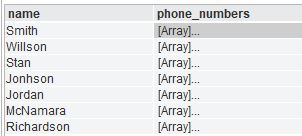phone_array_table view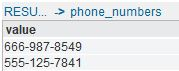phone_numbers values

We want to transform the two phone_numbers rows into two columns, home_phone_number and office_phone_number.

To do this, we apply the pivotregister custom function over the phone_numbers column, so it returns a record with the properties home_phone_number and office_phone_number and the values of the phone_numbers column:

create or replace view phone_table_aux as select name, pivotregister(phone_numbers, {ROW ('home_phone_number'), ROW ('office_phone_number')}) from phone_array_table;

Or

create or replace view phone_table_aux as select name, pivotregister(phone_numbers, 'home_phone_number:string,office_phone_number:string') from phone_array_table;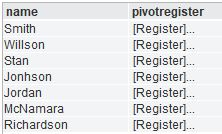phone_table_aux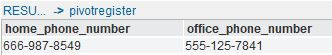pivot register result value

Finally, we can created a derived view over phone_table_aux and project the fields of the pivotregister record the view, and we will get the pivot result: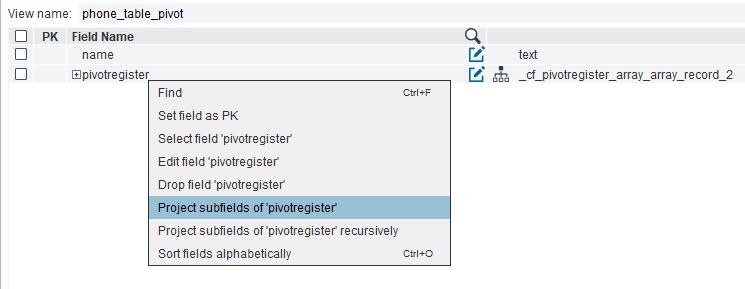Project subfields option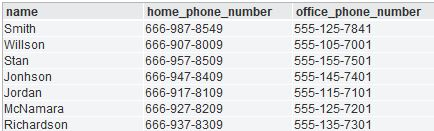phone_array_table after pivot

This function can be used to transform JSONs data sources, created for example from Google Sheets or Google Analytics sources, in tables. In this case, it may be possible that the first element of the JSON was the header of the table. If we want to specify the column types, the first tuple might be null, since the header has text values.

Example:

We want to transform this JSON in a table with six columns:

• Date of type timestampz
• C1 of type boolean
• C2 of type double
• C3 of type time
• C4 of type integer
• C5 of type integer

{

"range": "'Sheet 1'!A1:E36",

"majorDimension": "ROWS",

"values": [

[

"Date",

"c1",

"c2",

"c3",

"c4",

"c5"

],

[

"2020-10-10T01:59:59+01:00",

"true",

"43.58",

"21:15:45",

"421",

"23"

],

[

"2020-10-11T01:59:59+01:00",

"true",

"43.79",

"21:15:45"

],

[

"2020-10-12T01:59:59+01:00",

"false",

"44.74",

"21:15:45"

],

[

"2020-10-13T01:59:59+01:00",

"true",

"45.10",

"21:15:45"

],

[

"2020-10-14T01:59:59+01:00",

"false",

"46.01",

"21:15:45",

"486",

"23"

]

]

}

After creating the datasource using this json we create the base view: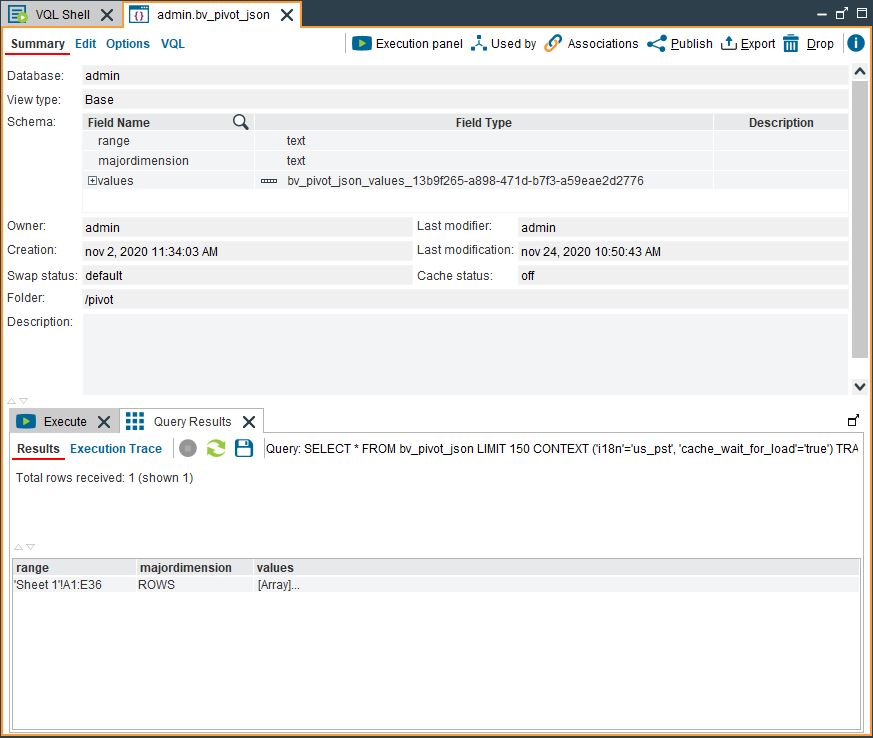Sample JSON base view

We need to create a derived view to flatten the values array: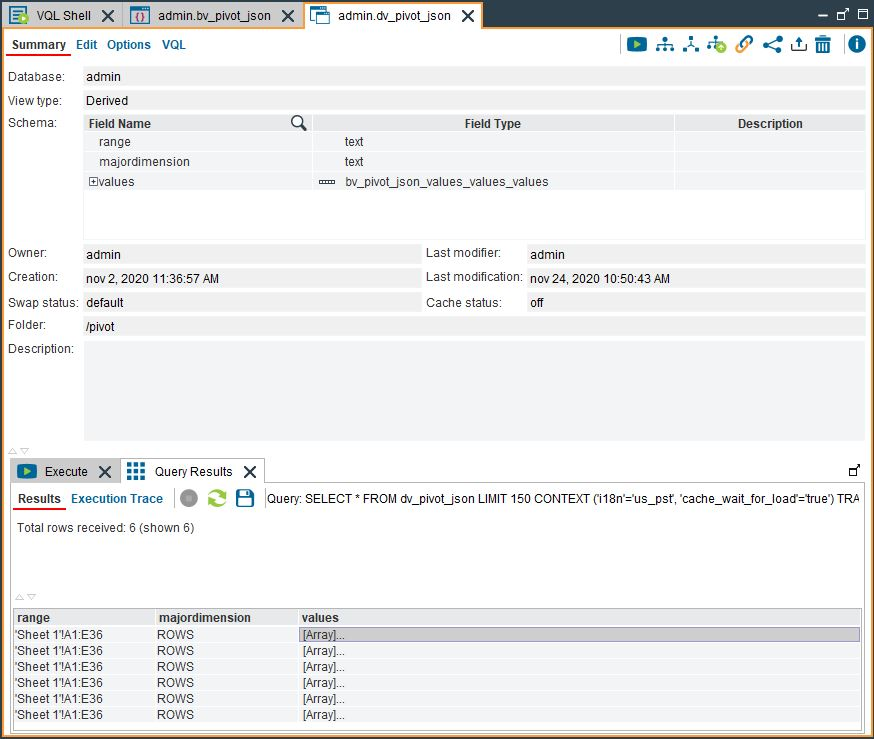Sample JSON derived view

In order to transform the array values in columns with the wanted types, we are going to apply the pivotregister function:

create or replace view pivot_json_aux as select pivotregister(values, 'Date:timestampz, C1:boolean, C2:double, C3:time, C4:long, C5:integer') from dv_pivot_json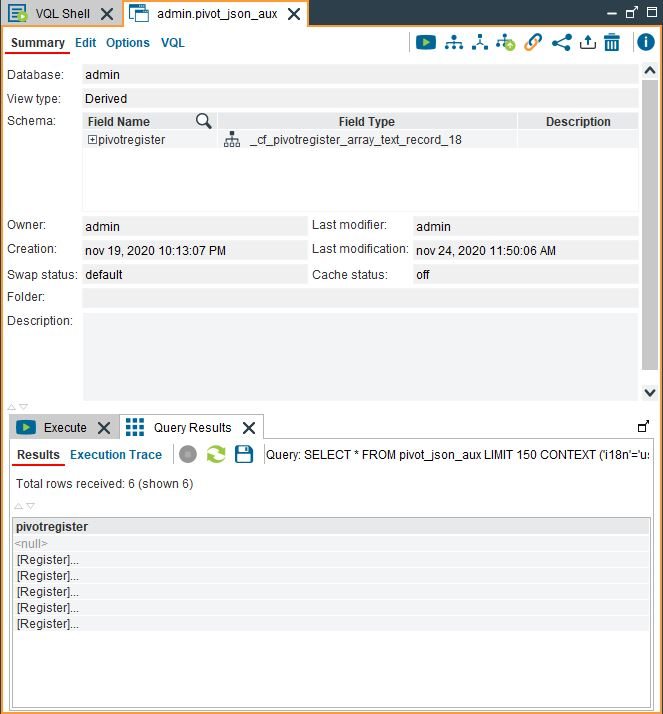Sample JSON view after pivot rows

To avoid having a null row, we can number the rows of the table. To do this, we have to add a new column, rownum, to the view in which the record subfields are projected. This new column is a call to the rownum() function:Add new field option in VPD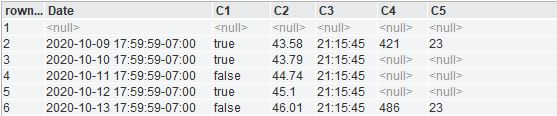Pivot table with rownum

Now, we just have to create a derived view which removes the first row. To do this, we need to add the following where condition: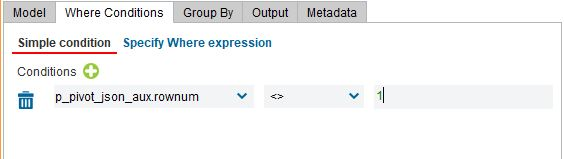Where condition to filter first row

Remember to remove the rownum field in the Output of the derived view and we will have the result view: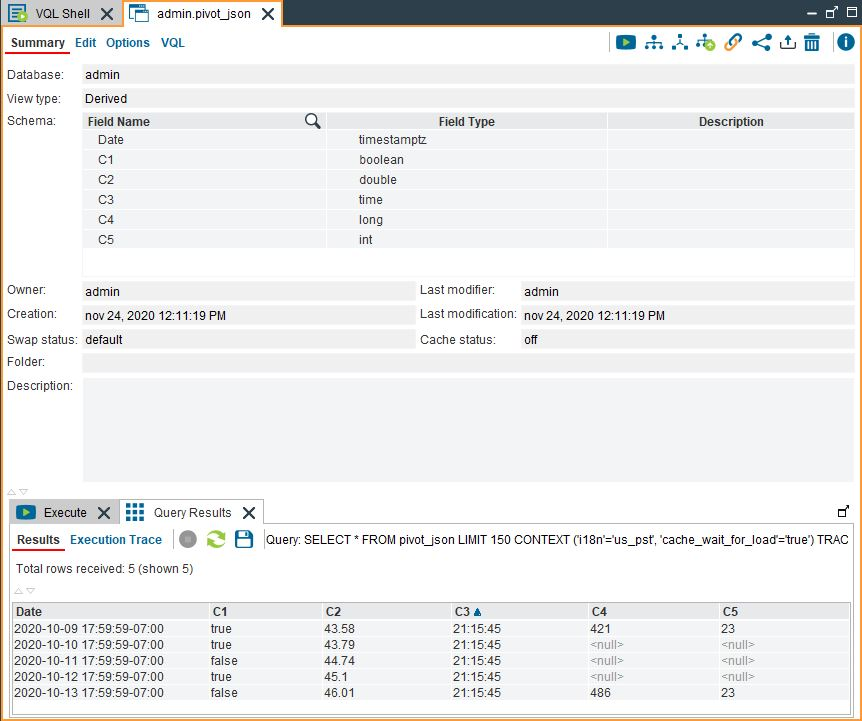Pivot table result

### unpivot

• unpivotregister(record): This function transforms, in the provided record, columns to rows.

For example:

We want to unpivot the firstname and lastname of the actor table.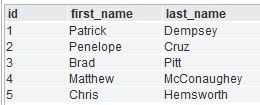actor table

To do this, we create a register, and then apply the custom function unpivotregister, so it returns the array of key, value with column_name, register_value:

create or replace view unpivot_actor_aux as select id, unpivotregister(register(first_name, last_name)) from actor;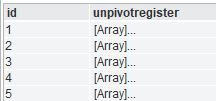unpivot_actor_aux view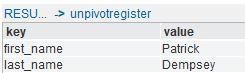unpivot register value

Finally, we can flatten the view, and we will get the unpivot result:

select id, key, value from flatten unpivot_actor_aux as a (a.unpivotregister);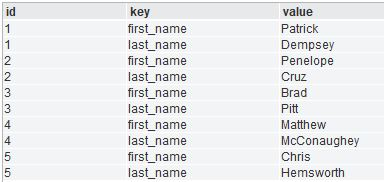unpivot actor table